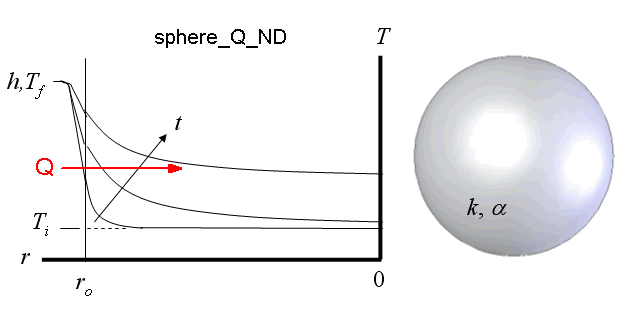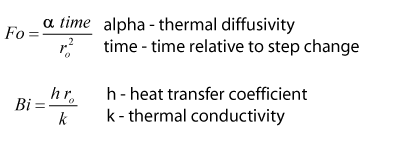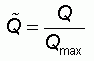Contents - Index

Sphere_Q_NDThe function sphere_Q_ND(Fo, Bi) returns the non-dimensional heat transfer into a sphere whose outer surface (r=r_o) is exposed to convection. The supplied values, Fo, Bi represent Fourier number and Biot number respectively.  See Section 3.5.2 of Nellis and Klein for more information.

Inputs:Output:Example:
r_bar=0.3
Fo=0.23
Bi=1.02
Q_hat=sphere_Q_ND(Fo, Bi)

{Solution: Q_hat=0.4466}

Transient Conduction Index